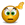# Functions on Filtered Data

## Excel Help for Functions On Filtered Data in Analyzing Corporate Financial Data

### Functions On Filtered Data

Rate this:The SUBTOTAL function provides some basic calculations on filtered data such as averages, standard deviation, maximum and minimum values. More advanced calculations such as statistical functions including the CORREL correlation function as well as any of the other inbuilt functions within Excel cannot be performed on filtered data ranges without applying complicated array formulas.

Another alternative and effective solution is to apply a user defined function to the filtered data ranges in order to return an array of values representing only the visible data. This way all functions in Excel can be applied to filtered data including common statistical functions such as correlations and forecasting.

The following VBA code creates a user defined function that returns only the visible data with the input range.

VBA Code
 Function VISIBLE(InputRange As Range) As VariantDim arrOut(), ic As VariantDim vcount As Longvcount = 0For Each ic In InputRange  If ic.Rows.Hidden = False And ic.Columns.Hidden = False Then    ReDim Preserve arrOut(vcount)    If IsNumeric(ic.Value) Then      arrOut(vcount) = ic.Value    Else      arrOut(vcount) = 0    End If    vcount = vcount + 1  End IfNextVISIBLE = arrOutEnd Function

This can then be used within standard Excel functions on the filtered data such as correlation in

=CORREL(VISIBLE(A2:A100),VISIBLE(B2:B100))